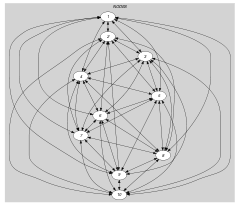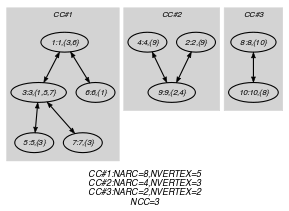## 5.329. proper_forest

Origin
Constraint

$\mathrm{𝚙𝚛𝚘𝚙𝚎𝚛}_\mathrm{𝚏𝚘𝚛𝚎𝚜𝚝}\left(\mathrm{𝙽𝚃𝚁𝙴𝙴𝚂},\mathrm{𝙽𝙾𝙳𝙴𝚂}\right)$

Arguments
 $\mathrm{𝙽𝚃𝚁𝙴𝙴𝚂}$ $\mathrm{𝚍𝚟𝚊𝚛}$ $\mathrm{𝙽𝙾𝙳𝙴𝚂}$ $\mathrm{𝚌𝚘𝚕𝚕𝚎𝚌𝚝𝚒𝚘𝚗}\left(\mathrm{𝚒𝚗𝚍𝚎𝚡}-\mathrm{𝚒𝚗𝚝},\mathrm{𝚗𝚎𝚒𝚐𝚑𝚋𝚘𝚞𝚛}-\mathrm{𝚜𝚟𝚊𝚛}\right)$
Restrictions
 $\mathrm{𝙽𝚃𝚁𝙴𝙴𝚂}\ge 0$ $\mathrm{𝚛𝚎𝚚𝚞𝚒𝚛𝚎𝚍}$$\left(\mathrm{𝙽𝙾𝙳𝙴𝚂},\left[\mathrm{𝚒𝚗𝚍𝚎𝚡},\mathrm{𝚗𝚎𝚒𝚐𝚑𝚋𝚘𝚞𝚛}\right]\right)$ $|\mathrm{𝙽𝙾𝙳𝙴𝚂}|\mathrm{mod}2=0$ $\mathrm{𝙽𝙾𝙳𝙴𝚂}.\mathrm{𝚒𝚗𝚍𝚎𝚡}\ge 1$ $\mathrm{𝙽𝙾𝙳𝙴𝚂}.\mathrm{𝚒𝚗𝚍𝚎𝚡}\le |\mathrm{𝙽𝙾𝙳𝙴𝚂}|$ $\mathrm{𝚍𝚒𝚜𝚝𝚒𝚗𝚌𝚝}$$\left(\mathrm{𝙽𝙾𝙳𝙴𝚂},\mathrm{𝚒𝚗𝚍𝚎𝚡}\right)$ $\mathrm{𝙽𝙾𝙳𝙴𝚂}.\mathrm{𝚗𝚎𝚒𝚐𝚑𝚋𝚘𝚞𝚛}\ge 1$ $\mathrm{𝙽𝙾𝙳𝙴𝚂}.\mathrm{𝚗𝚎𝚒𝚐𝚑𝚋𝚘𝚞𝚛}\le |\mathrm{𝙽𝙾𝙳𝙴𝚂}|$ $\mathrm{𝙽𝙾𝙳𝙴𝚂}.\mathrm{𝚗𝚎𝚒𝚐𝚑𝚋𝚘𝚞𝚛}\ne \mathrm{𝙽𝙾𝙳𝙴𝚂}.\mathrm{𝚒𝚗𝚍𝚎𝚡}$
Purpose

Cover an undirected graph $G$ by a set of $\mathrm{𝙽𝚃𝚁𝙴𝙴𝚂}$ trees (i.e., a tree is a connected graph without cycles that contains at least two vertices [Cayley89]) in such a way that each vertex of $G$ belongs to one distinct tree.

Example
$\left(\begin{array}{c}3,〈\begin{array}{cc}\mathrm{𝚒𝚗𝚍𝚎𝚡}-1\hfill & \mathrm{𝚗𝚎𝚒𝚐𝚑𝚋𝚘𝚞𝚛}-\left\{3,6\right\},\hfill \\ \mathrm{𝚒𝚗𝚍𝚎𝚡}-2\hfill & \mathrm{𝚗𝚎𝚒𝚐𝚑𝚋𝚘𝚞𝚛}-\left\{9\right\},\hfill \\ \mathrm{𝚒𝚗𝚍𝚎𝚡}-3\hfill & \mathrm{𝚗𝚎𝚒𝚐𝚑𝚋𝚘𝚞𝚛}-\left\{1,5,7\right\},\hfill \\ \mathrm{𝚒𝚗𝚍𝚎𝚡}-4\hfill & \mathrm{𝚗𝚎𝚒𝚐𝚑𝚋𝚘𝚞𝚛}-\left\{9\right\},\hfill \\ \mathrm{𝚒𝚗𝚍𝚎𝚡}-5\hfill & \mathrm{𝚗𝚎𝚒𝚐𝚑𝚋𝚘𝚞𝚛}-\left\{3\right\},\hfill \\ \mathrm{𝚒𝚗𝚍𝚎𝚡}-6\hfill & \mathrm{𝚗𝚎𝚒𝚐𝚑𝚋𝚘𝚞𝚛}-\left\{1\right\},\hfill \\ \mathrm{𝚒𝚗𝚍𝚎𝚡}-7\hfill & \mathrm{𝚗𝚎𝚒𝚐𝚑𝚋𝚘𝚞𝚛}-\left\{3\right\},\hfill \\ \mathrm{𝚒𝚗𝚍𝚎𝚡}-8\hfill & \mathrm{𝚗𝚎𝚒𝚐𝚑𝚋𝚘𝚞𝚛}-\left\{10\right\},\hfill \\ \mathrm{𝚒𝚗𝚍𝚎𝚡}-9\hfill & \mathrm{𝚗𝚎𝚒𝚐𝚑𝚋𝚘𝚞𝚛}-\left\{2,4\right\},\hfill \\ \mathrm{𝚒𝚗𝚍𝚎𝚡}-10\hfill & \mathrm{𝚗𝚎𝚒𝚐𝚑𝚋𝚘𝚞𝚛}-\left\{8\right\}\hfill \end{array}〉\hfill \end{array}\right)$

The $\mathrm{𝚙𝚛𝚘𝚙𝚎𝚛}_\mathrm{𝚏𝚘𝚛𝚎𝚜𝚝}$ constraint holds since the undirected graph associated with the items of the $\mathrm{𝙽𝙾𝙳𝙴𝚂}$ collection corresponds to a forest containing $\mathrm{𝙽𝚃𝚁𝙴𝙴𝚂}=3$ trees: each tree respectively involves the vertices $\left\{1,3,5,6,7\right\}$, $\left\{2,4,9\right\}$ and $\left\{8,10\right\}$.

Typical
 $\mathrm{𝙽𝚃𝚁𝙴𝙴𝚂}>0$ $|\mathrm{𝙽𝙾𝙳𝙴𝚂}|>1$
Symmetry

Items of $\mathrm{𝙽𝙾𝙳𝙴𝚂}$ are permutable.

Arg. properties

Functional dependency: $\mathrm{𝙽𝚃𝚁𝙴𝙴𝚂}$ determined by $\mathrm{𝙽𝙾𝙳𝙴𝚂}$.

Algorithm

A filtering algorithm for the $\mathrm{𝚙𝚛𝚘𝚙𝚎𝚛}_\mathrm{𝚏𝚘𝚛𝚎𝚜𝚝}$ constraint was proposed by N. Beldiceanu et al. in [BeldiceanuKatrielLorca06]. It achieves hybrid-consistency and its running time is dominated by the complexity of finding all edges that do not belong to any maximum cardinality matching in an undirected $n$-vertex, $m$-edge graph, i.e., $O\left(m·n\right)$.

Systems

tree in Choco.

Keywords
Arc input(s)

$\mathrm{𝙽𝙾𝙳𝙴𝚂}$

Arc generator
$\mathrm{𝐶𝐿𝐼𝑄𝑈𝐸}$$\left(\ne \right)↦\mathrm{𝚌𝚘𝚕𝚕𝚎𝚌𝚝𝚒𝚘𝚗}\left(\mathrm{𝚗𝚘𝚍𝚎𝚜}\mathtt{1},\mathrm{𝚗𝚘𝚍𝚎𝚜}\mathtt{2}\right)$

Arc arity
Arc constraint(s)
$\mathrm{𝚒𝚗}_\mathrm{𝚜𝚎𝚝}$$\left(\mathrm{𝚗𝚘𝚍𝚎𝚜}\mathtt{2}.\mathrm{𝚒𝚗𝚍𝚎𝚡},\mathrm{𝚗𝚘𝚍𝚎𝚜}\mathtt{1}.\mathrm{𝚗𝚎𝚒𝚐𝚑𝚋𝚘𝚞𝚛}\right)$
Graph property(ies)
 $•$$\mathrm{𝐍𝐕𝐄𝐑𝐓𝐄𝐗}$$=\left($$\mathrm{𝐍𝐀𝐑𝐂}$$+2*\mathrm{𝙽𝚃𝚁𝙴𝙴𝚂}\right)/2$ $•$$\mathrm{𝐍𝐂𝐂}$$=\mathrm{𝙽𝚃𝚁𝙴𝙴𝚂}$ $•$$\mathrm{𝐍𝐕𝐄𝐑𝐓𝐄𝐗}$$=|\mathrm{𝙽𝙾𝙳𝙴𝚂}|$

Graph class
$\mathrm{𝚂𝚈𝙼𝙼𝙴𝚃𝚁𝙸𝙲}$

Graph model

The graph constraint forces the following conditions:

• Each connected component of the final graph has $n$ vertices and $2·\left(n-1\right)$ arcs. This is equivalent to the fact that each connected component has not any cycle.

• Since we use the $\mathrm{𝐶𝐿𝐼𝑄𝑈𝐸}\left(\ne \right)$ arc-generator and since, by definition, the final graph does not contain any isolated vertex, each connected component of the final graph involves more than one vertex.

• The number of connected components of the final graph is equal to $\mathrm{𝐍𝐂𝐂}$.

• All the vertices of the initial graph belong to the final graph.

• The final graph is symmetric.

Parts (A) and (B) of Figure 5.329.1 respectively show the initial and final graph associated with the Example slot. For each connected component we display its number of arcs as well as its number of vertices. The $\mathrm{𝚙𝚛𝚘𝚙𝚎𝚛}_\mathrm{𝚏𝚘𝚛𝚎𝚜𝚝}$ constraint holds since the final graph has $\mathrm{𝙽𝚃𝚁𝙴𝙴𝚂}=\mathrm{𝐍𝐂𝐂}=3$ connected components and no cycle.

##### Figure 5.329.1. Initial and final graph of the $\mathrm{𝚙𝚛𝚘𝚙𝚎𝚛}_\mathrm{𝚏𝚘𝚛𝚎𝚜𝚝}$ constraint(a)(b)